07 Nov

So maybe you’ve made the classic six-strut tensegrity (or perhaps you’ve just looked at the pictures) and you’re wondering what shape that is, exactly. Naturally, since mathematics is among other things the science of shape and structure, understanding that is going to involve a little math. And in mathematics, sometimes it’s easiest to understand something by breaking it down into the pieces that are there, but other times it’s actually easier to understand something by adding (or pretending to add) pieces that aren’t there.

So take a look again at the structure you built.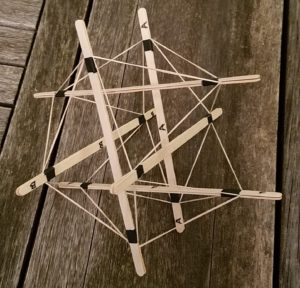Notice that in many cases, two neighboring endpoints of struts are connected by segments of rubber band. However, in other cases, there are endpoints of two struts that are close together but are not connected at all. So let’s imagine what the structure would look like if all of the nearest neighbors were connected.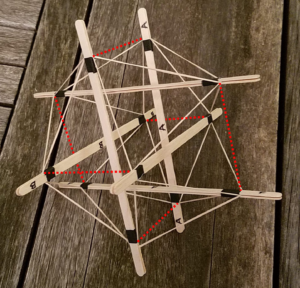Now, if we look at the outer surface of the structure, we see a much simpler pattern. Every outermost flat shape of the structure — which we call a face of the structure — is a triangle. There are 20 of these triangles, and at each endpoint of a strut, which makes a sort of “corner” of the structure called a vertex, exactly five of the triangles meet. There’s just one geometric shape fitting those facts, called the icosahedron.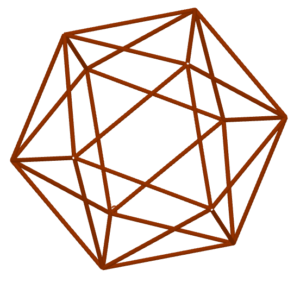This shape is simple and natural enough to have been discovered at least two and a half millennia ago, as one of the five Platonic Solids of the ancient Greeks. These are the shapes with identical regular polygonal faces and identical vertices, and it is a remarkable and beautiful fact that only five different Platonic solids exist. Yet the icosahedron is also intriguing and pleasing enough that it’s still of interest today.

One of the most important characteristics of the icosahedron is that it has very many symmetries. A symmetry of an object is some transformation, like a rotation or a translation or some combination thereof, which brings the shape back to coincide with itself. For example, if we were to rotate the icosahedron one-fifth of a turn around the axis indicated by the dotted blue line in this diagram, it would again look exactly the same, even though we moved it.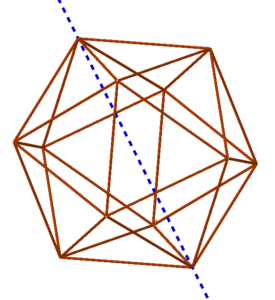In the idealized world of this Platonic icosahedron, how can we represent the struts? Each one of them connects two of the vertices of the icosahedron, so it is what you might call a diagonal of the icosahedron. Let’s draw all six of them on our diagram.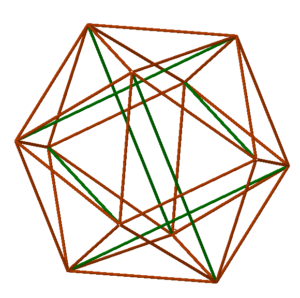Note how these diagonals are arranged; each one connects a vertex to another vertex that’s not adjacent, but lies “two edges away” from the starting vertex. They come in three pairs of parallel diagonals. Moreover, all six diagonals are “equivalent” under the symmetries of the icosahedron, which means to say that there’s a symmetry of the icosahedron which moves any of the struts to the position of any one of the other struts. That corresponds with the fact that all of our struts are the same length: in order to get an icosahedron, it’s necessary to use struts that are the same length, because there’s no way to tell one strut from another. They’re all interchangeable by the symmetries of the icosahedron.

We’ll close this tour of the math underlying the six-strut tensegrity with a perhaps inconvenient-seeming observation. If you look closely at the structure you’ve built (or at the pictures above), you will see that the icosahedron is not actually perfectly regular. In the diagram, the dotted sides of the triangles are not the same lengths as the rubber-band sides of the triangles. So our tensegrity structure is not actually a Platonic regular icosahedron; you might instead dub it an “icloseahedron” since it’s really close to, but not quite identical to, the idealized structure we envisioned.

But why isn’t this structure regular? After all, we built it in a very symmetric way. Well, there’s one way in which the physical structure of the six-strut tensegrity differs markedly from a true icosahedron — and we’ll take that up in the next MathStream article.

HERE!! go on about symmetries, show how the struts relate, point out that the tensegrity isn’t precisely a regular icosahedron, end with wondering why, saying that will have to wait for another post.